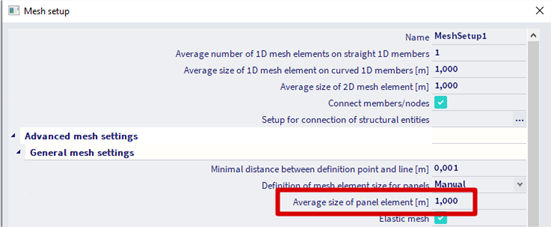# Load transfer method on panel

## Details of Load transfer method on panel

Different methods are available for the loads transfer via load panels :

Which one to choose?

By default, the method is « Tributary area » : loads are distributed according to the break lines.

But this method is available only with uniform surface loads on the whole panel.

In case of partial surface loads on a panel, or triangular loads, or trapezoidal loads, …, a warning will be displayed because we need to use another method.

## Standard

The load is distributed according to factors.

In the above example, the load would be distributed by 1/3, 1/3, 1/3 on each bar, because factor 1 is defined on each bar (even if the load is a linear or a point load in a corner of the panel).

The distribution doesn’t take into account the loads locations, but rather the defined factors.

This method is less used than the others.

## Accurate (FEM) - all load transfer directions

When you use a load panel with the Load transfer method “Accurate (FEM), following analysis is performed (see also our helppage FEM method):

The solver uses the mesh setting 'Average size of panel elements [m]':For the FEM load transfer methods, there are options 'hinged support' and 'fixed support'. The distribution of loads is done following the indicated, giving for example the following results:

The analysis is done with fictive 2D elements with the following parameters:

• E modulus = 1 GPa
• Poisson coefficient = 0.25

## Accurate (FEM) - 1 direction

If the load transfer direction X and Y are set differently, for example 80% and 20%, then the parameters of the orthotropy are calculated proportionally.

For load transfer direction set to X or Y, the E1:E2 ratio is taken as 80:20% and E = E1 + E2 = 100%.

• D11 and D44 = 80%
• D22 and D55 = 20%
• D12 and D33 = 50%

## Example

### Accurate (FEM), fixed link with beams

This method is close to the tributary areas method, because the distribution is divided by 2 on each side.

### Accurate (FEM), hinged link with beams

Let’s have a look at the simple example below:

Let’s apply a surface horizontal load on each panel.

Results are in line with the previous description of this option: